Courses

# NCERT Solutions(Part - 1) - Perimeter and Area Class 7 Notes | EduRev

## Class 7: NCERT Solutions(Part - 1) - Perimeter and Area Class 7 Notes | EduRev

The document NCERT Solutions(Part - 1) - Perimeter and Area Class 7 Notes | EduRev is a part of the Class 7 Course Mathematics (Maths) Class 7.
All you need of Class 7 at this link: Class 7

EXERCISE 11.1
Question 1: The length and breadth of a rectangular piece of land are 500 m and 300 m respectively. Find:
(i) Its area.
(ii) The cost of the land, if 1 m2 of the land costs Rs 10,000.
Solution:
From the question it is given that,
Length of the rectangular piece of land = 500 m
Breadth of the rectangular piece of land = 300 m
Then,
(i) Area of rectangle = Length × Breadth
= 500 × 300
= 15,0000 m2
(ii) Cost of the land for 1 m2 = Rs. 10000
Cost of the land for 150000 m2 = 10000 × 150000
= Rs. 15,00000000

Question 2: Find the area of a square park whose perimeter is 320 m.
Solution:
From the question it is given that,
Perimeter of the square park = 320 m
4 × Length of the side of park = 320 m
Then,
Length of the side of park = 320/4 = 80 m
So, Area of the square park = (length of the side of park)2 = (80)2 m2 = 6400 m2

Question 3: Find the breadth of a rectangular plot of land, if its area is 440 m2 and the length is 22 m. Also find its perimeter.
Solution:
Area of rectangular park = 440 m2
⇒ length x breadth = 440 m2
⇒  22 x breadth = 440
⇒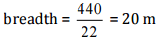Now, Perimeter of rectangular park
= 2 [22 + 20]
= 2 x 42 = 84 m
Thus, the perimeter of rectangular park is 84 m.

Question 4: The perimeter of a rectangular sheet is 100 cm. If the length is 35 cm, find its breadth. Also find the area.
Solution:
Perimeter of the rectangular sheet = 100 cm
⇒ 2 (length + breadth) = 100 cm
⇒ 2 (35 + breadth) = 100
⇒ 35 + breadth = 100/2
⇒ 35 + breadth = 50
⇒ breadth = 50 - 35
Now, Area of rectangular sheet = length x breadth
= 35 x 15 = 525 cm2
Thus, breadth and area of rectangular sheet are 15 cm and 525 cm2 respectively.

Question 5: The area of a square park is the same as a rectangular park. If the side of the square park is 60 m and the length of the rectangular park is 90 cm, find the breadth of the rectangular park.
Solution:
Given:
The side of the square park = 60 m
The length of the rectangular park = 90 m
According to the question,
Area of square park = Area of rectangular park
⇒ side x side = length x breadth
⇒ 60 x 60 = 90 x breadth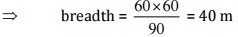Thus, the breadth of the rectangular park is 40 m.

Question 6: A wire is in the shape of a rectangle. Its length is 40 cm and breadth is 22 cm. If the same wire is rebent in the shape of a square, what will be the measure of each side. Also find which shape encloses more area?
Solution:
According to the question,
Perimeter of square = Perimeter of rectangle
⇒ 4 x side = 2 (length + breadth)
⇒ 4 x side = 2 (40 + 22)
⇒ 4xside = 2x62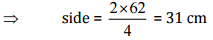Thus, the side of the square is 31 cm.
Now, Area of rectangle = length x breadth = 40x22 = 880 cm2
And Area of square = side x side = 31 x 31 = 961 cm2
Therefore, on comparing, the area of square is greater than that of rectangle.

Question 7: The perimeter of a rectangle is 130 cm. If the breadth of the rectangle is 30 cm, find its length. Also, find the area of the rectangle.
Solution:
Perimeter of rectangle = 130 cm
⇒ 2 (length + breadth) = 130 cm
⇒ 2 (length + 30) = 130
⇒ length + 30 = 130/2
⇒ length+ 30 = 65
⇒ length = 65 - 30 = 35 cm
Now area of rectangle = length x breadth = 35 x 30 = 1050 cm2
Thus, the area of rectangle is 1050 cm2.

Question 8: A door of length 2 m and breadth 1 m is fitted in a wall. The length of the wall is 4.5 m and the breadth is 3.6 m. Find the cost of white washing the wall, if the rate of white washing the wall is Rs 20 per m2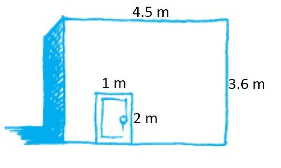Solution:
Area of rectangular door = length x breadth = 2 m x 1 m = 2 m2
Area of wall including door = length x breadth = 4.5 m x 3.6 m = 16.2 m2
Now, Area of wall excluding door = Area of wall including door - Area of door = 16.2-2 = 14.2 m2
Since, the rate of white washing of 1 m2 the wall = Rs 20
Therefore, the rate of white washing of 14.2 m2 the wall = 20 x 14.2 = Rs 284
Thus, the cost of white washing the wall excluding the door is Rs 284.

EXERCISE 11.2
Question 1: Find the area of each of the following parallelograms: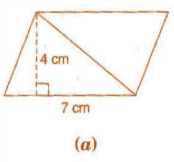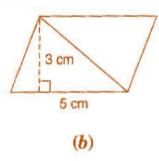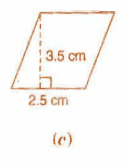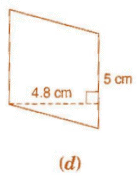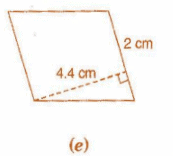Solution:
We know that the area of parallelogram = base x height
(a) Here base = 7 cm and height = 4 cm
∴ Area of parallelogram = 7x4 = 28 cm2
(b) Here base = 5 cm and height = 3 cm
∴ Area of parallelogram = 5x3 = 15 cm2
(c) Here base = 2.5 cm and height = 3.5 cm
∴ Area of parallelogram = 2.5 x 3.5 = 8.75 cm2

(d) Here base = 5 cm and height = 4.8 cm
∴ Area of parallelogram = 5 x 4.8 = 24 cm2
(e) Here base = 2 cm and height = 4.4 cm
∴ Area of parallelogram = 2 x 4.4 = 8.8 cm2

Question 2: Find the area of each of the following triangles: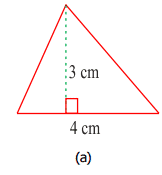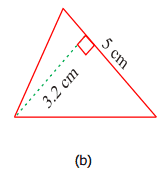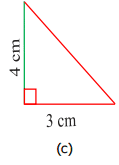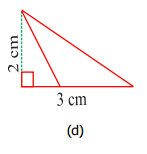Solution:
We know that the area of triangle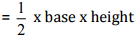(a) Here, base = 4 cm and height = 3 cm
∴ Area of triangle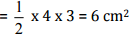(b) Here, base = 5 cm and height = 3.2 cm
∴ Area of triangle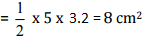(c) Here, base = 3 cm and height = 4 cm
∴ Area of triangle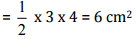(d) Here, base = 3 cm and height = 2 cm
∴ Area of triangle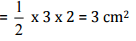Question 3: Find the missing values:

 S. No. Base Height Area of the parallelogram a. 20 cm 246 cm2 b. 15 cm 154.5 cm2 c. 84 cm 48.72 cm2 d. 15.6 cm 16.38 cm2

Solution:
We know that the area of parallelogram = base x height
(a) Here, base = 20 cm and area = 246 cm2
∴ Area of parallelogram = base x height
⇒ 246 = 20 x height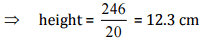(b) Here, height = 15 cm and area = 154.5 cm2
∴ Area of parallelogram = base x height
⇒ 154.5 = base x 15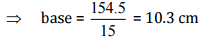(c) Here, height = 8.4 cm and area = 48.72 cm2
∴ Area of parallelogram = base x height
⇒ 48.72 = base x 8.4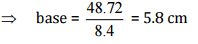(d) Here, base = 15.6 cm and area = 16.38 cm2
∴ Area of parallelogram = base x height
⇒ 16.38 = 15.6 x height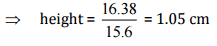Thus, the missing values are:

 S. No. Base Height Area of the parallelogram a. 20 cm 12.3 cm 246 cm2 b. 10.3 cm 15 cm 154.5 cm2 c. 5.8 cm 84 cm 48.72 cm2 d. 15.6 cm 1.05 16.38 cm2

Question 4: Find the missing values:

 Base Height Area of triangle 15 cm ------ 87 cm2 -------- 31.4 mm 1256 mm2 22 cm ------ 170.5 cm2

Solution:
We know that the area of triangle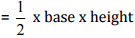In first row, base = 15 cm and area = 87 cm2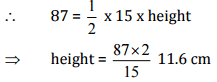In second row, height = 31.4 mm and area = 1256 mm2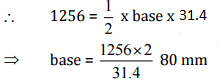In third row, base = 22 cm and area = 170.5 cm2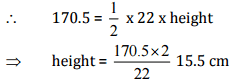Thus, the missing values are:

 Base Height Area of triangle 15 cm 11.6 cm 87 cm2 80 mm 31.4 mm 1256 mm2 22 cm 15.5 cm 170.5 cm2

Question 5: PQRS is a parallelogram (Fig 11.23), QM is the height from Q to SR and QN is the height from Q to PS. If SR = 12 cm and QM = 7.6 cm. Find:
(a) the area of the parallelogram PQRS
(b) QN, if PS = 8 cm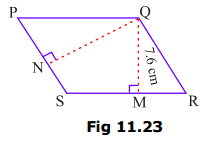Solution:
Given:
SR = 12 cm, QM = 7.6 cm, PS = 8 cm,
(a) Area of parallelogram = base x height
= 12 x 7.6 = 91.2 cm2
(b) Area of parallelogram = base x height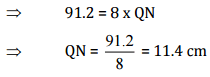Question 6: DL and BM are the heights on sides AB and AD respectively of parallelogram ABCD (Fig 11.24). If the area of the parallelogram is 1470 cm2, AB = 35 cm and AD = 49 cm, Find the length of BM and DL.Solution:
Given:
Area of parallelogram = 1470 cm2
Base (AB) = 35 cm and base (AD) = 49 cm
Since Area of parallelogram = base x height
⇒ 1470 = 35 x DL
⇒ DL = 1470/35
⇒ DL = 42 cm
Again, Area of parallelogram = base x height
⇒ 1470 = 49 x BM
⇒ BM  = 1470/49
⇒ BM = 30 cm
Thus, the lengths of DL and BM are 42 cm and 30 cm respectively.

Question 7: Δ ABC is right angled at A (Fig 11.25). AD is perpendicular to BC. If AB = 5 cm, BC = 13 cm and AC = 12 cm, find the area of Δ ABC. Also, find the length of AD.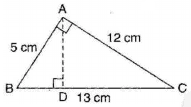Solution:Question 8: ΔABC is isosceles with AB = AC = 7.5 cm and BC = 9 cm (Fig 11.26). The height AD from A to BC, is 6 cm. Find the area of ΔABC. What will be the height from C to AB i.e., CE?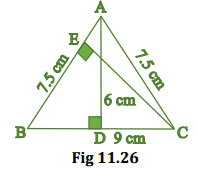Solution:
In ΔABC, AD = 6 cm and BC = 9 cm
Area of triangle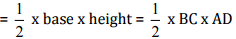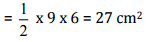Again, Area of triangle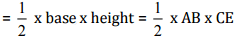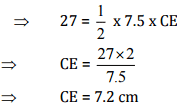Thus, height from C to AB i.e., CE is 7.2 cm.

The document NCERT Solutions(Part - 1) - Perimeter and Area Class 7 Notes | EduRev is a part of the Class 7 Course Mathematics (Maths) Class 7.
All you need of Class 7 at this link: Class 7Use Code STAYHOME200 and get INR 200 additional OFF Use Coupon Code
All Tests, Videos & Notes of Class 7: Class 7

### Top Courses for Class 7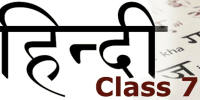## Mathematics (Maths) Class 7

161 videos|237 docs|45 tests

### Top Courses for Class 7Track your progress, build streaks, highlight & save important lessons and more!

,

,

,

,

,

,

,

,

,

,

,

,

,

,

,

,

,

,

,

,

,

;